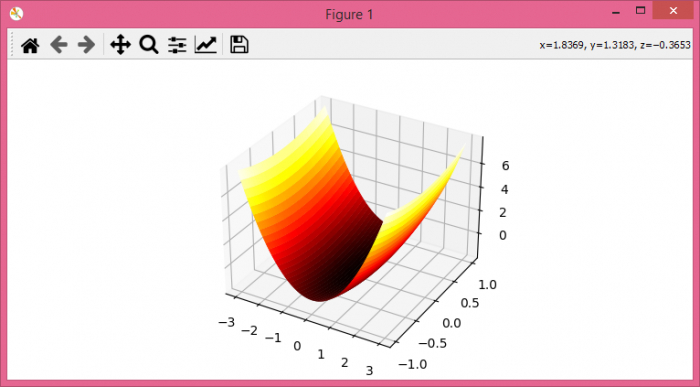# How to obtain 3D colored surface via Python?

To obtain 3D colored surface via Python, we can take the following steps −

## Steps

• Set the figure size and adjust the padding between and around the subplots.

• Create x and y data points using numpy.

• Get 3D data, i.e., z.

• Create a new figure or activate an existing figure.

• Get the 3D axes.

• Create a surface plot.

• To display the figure, use show() method.

## Example

import numpy as np
import matplotlib.pyplot as plt

plt.rcParams["figure.figsize"] = [7.50, 3.50]
plt.rcParams["figure.autolayout"] = True

x = np.linspace(-3, 3, 100)
y = np.cos(x)

x, y = np.meshgrid(x, y)

z = x ** 2 + y ** 2 - 2

fig = plt.figure()

ax = plt.axes(projection='3d')

surf = ax.plot_surface(x, y, z,
cmap=plt.get_cmap('hot'),
edgecolor='none')

plt.show()

## Output

It will produce the following output −